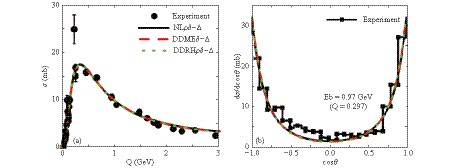# In-medium NN→NΔ cross section and its dependence on effective Lagrange parameters in isospin-asymmetric nuclear matter

• The in-medium $NN\rightarrow N\Delta$cross sections and its differential cross sections in isospin asymmetric nuclear medium are investigated in the framework of the one-boson exchange model by including isovector mesons, i.e., $\delta$and $\rho$mesons. Our results show that the in-medium $NN\rightarrow N\Delta$cross sections are suppressed when the density increases, and the differential cross sections become isotropic with an increase in the density around the $\Delta$threshold energy. The isospin splitting on the medium correction factor, $R=\sigma_{ NN\rightarrow N\Delta}^*/\sigma_{NN\rightarrow N\Delta}^{\rm{free}}$is observed for different channels of $NN\to N\Delta$, especially around the threshold energy for all the effective Lagrangian parameters. By analyzing the selected effective Lagrangian parameters, our results show that the larger effective mass is, the weaker medium correction $R$is.
••Get Citation
Ying Cui, Ying-Xun Zhang and Zhu-Xia Li. In-medium NN→NΔ cross section and its dependence on effective Lagrange parameters in isospin-asymmetric nuclear matter[J]. Chinese Physics C, 2019, 43(2): 1-1. doi: 10.1088/1674-1137/43/2/024105
Ying Cui, Ying-Xun Zhang and Zhu-Xia Li. In-medium NN→NΔ cross section and its dependence on effective Lagrange parameters in isospin-asymmetric nuclear matter[J]. Chinese Physics C, 2019, 43(2): 1-1.Milestone
Received: 2018-11-09
Article Metric

Article Views(229)
PDF Downloads(28)
Cited by(0)
Policy on re-use
To reuse of subscription content published by CPC, the users need to request permission from CPC, unless the content was published under an Open Access license which automatically permits that type of reuse.
###### 通讯作者: 陈斌, bchen63@163.com
• 1.

沈阳化工大学材料科学与工程学院 沈阳 110142

Title:
Email:

## In-medium NN→NΔ cross section and its dependence on effective Lagrange parameters in isospin-asymmetric nuclear matter

• 1. China Institute of Atomic Energy, Beijing 102413, China
• 2. Guangxi Key Laboratory Breeding Base of Nuclear Physics and Technology, Guangxi Normal University, Guilin 541004, China

Abstract: The in-medium $NN\rightarrow N\Delta$cross sections and its differential cross sections in isospin asymmetric nuclear medium are investigated in the framework of the one-boson exchange model by including isovector mesons, i.e., $\delta$and $\rho$mesons. Our results show that the in-medium $NN\rightarrow N\Delta$cross sections are suppressed when the density increases, and the differential cross sections become isotropic with an increase in the density around the $\Delta$threshold energy. The isospin splitting on the medium correction factor, $R=\sigma_{ NN\rightarrow N\Delta}^*/\sigma_{NN\rightarrow N\Delta}^{\rm{free}}$is observed for different channels of $NN\to N\Delta$, especially around the threshold energy for all the effective Lagrangian parameters. By analyzing the selected effective Lagrangian parameters, our results show that the larger effective mass is, the weaker medium correction $R$is.

### HTML2.   The model3.   Results and discussions
Reference (44)
PDF查看关注分享

Top

### 目录/DownLoad:  Full-Size Img  PowerPoint